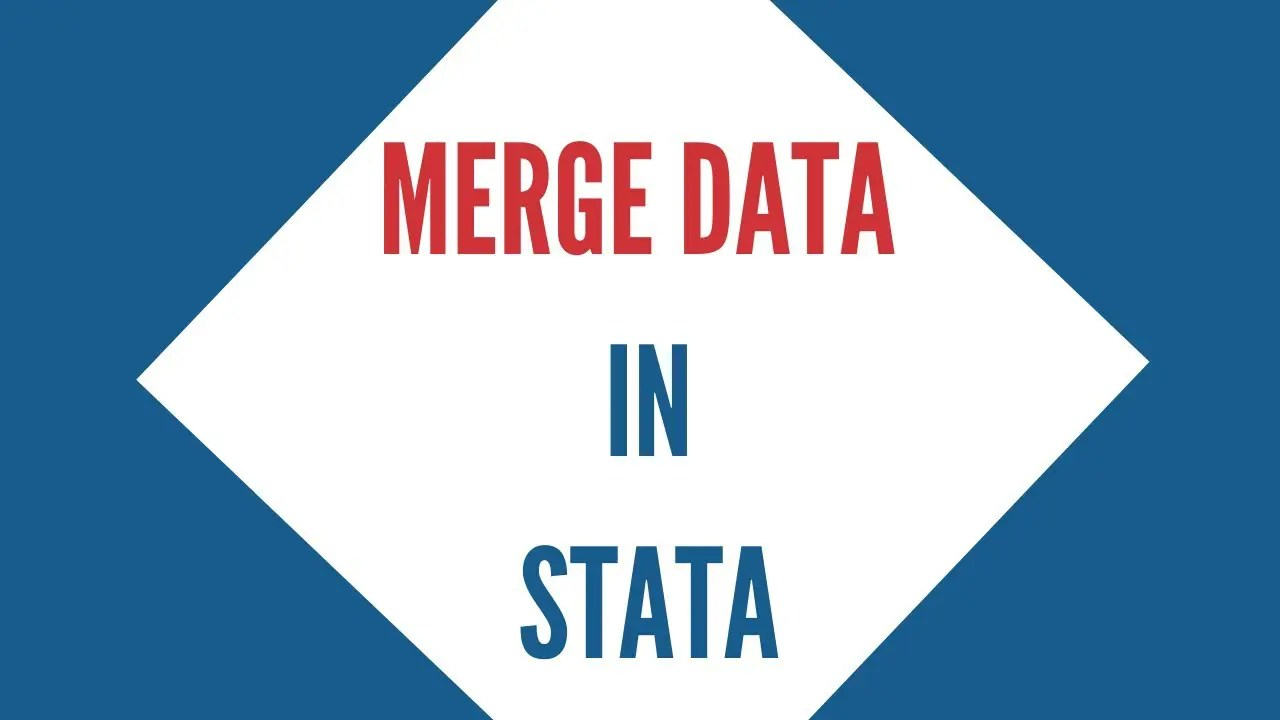## Nested Regression OR Hierarchical Regression in Stata

This article will go over how nested or hierarchical regressions are used in Stata. In many published academic papers, we see a single table representing results from various regression models run by the authors. Often, each subsequent regression adds one new variable to the list of covariates that an outcome variable is being regressed on. …

## Local and Global Macros in Stata

In a previous article, we discussed scalars and matrices in Stata, and how they help us extract results after an E-Class or R-Class command is run. This article will go over an associated topic – Macros. What Are Macros? Macros are somewhat similar to scalars: they store values. The pros and cons of using both …

## Extract Results From Analysis | E-Class and R-Class Commands

In this article, we will go over the difference between R-Class and E-Class commands and how we can access information through them. Whenever we execute a command in Stata, it stores the results in one of these commands. It is very common to use these commands to extract and use the result they store in …

## How to use Scalar and Matrix in Stata

This article goes over what scalars and matrices are and how to work with them in Stata. To illustrate the concepts, we will use Stata’s built in automobile dataset which can be loaded by using the following command: sysuse auto.dta, clear What Are Scalars and Matrices Scalars can be defined as placeholders in Stata that …## How to merge data in Stata | Combining datasets in Stata

Whenever you are working with more than one dataset, it is highly likely that you will need to combine them together to perform any analysis. In Stata, this is referred to as merging. There are different types of merges depending on the situation. Each type of merge is explained in its relevant sections. Many-to-one Merge …